# Pouillet’s Law

Pouillet ‘s Law is an important formula for the study of electrodynamics , it is a mathematical expression used to solve closed electrical circuits, formed by resistors generators and receivers .

This law, also known as Ohm’s law for closed circuits, allows calculating the electric current that flows through a loop of a circuit, and is defined as the ratio between the equivalent electromotive force and the equivalent resistance of the circuit.

## Definition of Pouillet’s law

Pouillet’s law is a simplification used to facilitate calculations involving simple electrical circuits . The results obtained by it can be verified by applying Kirchoff’s laws , which are generally used to solve slightly more complex electrical circuits, composed of two or more meshes.

Pouillet’s law is therefore a law of conservation of energy, it implies that the sum of all electrical potentials along a mesh must be zero, as stated by Kirchoff ‘s second law .

“The electromotive force of a generator is equal to the product of the current intensity and the total resistance of a circuit.”

Consider a real generator , with internal resistance i and electromotive force ε , whose terminals (A and B) are connected to an ohmic resistor of electrical resistance R, as shown in the figure: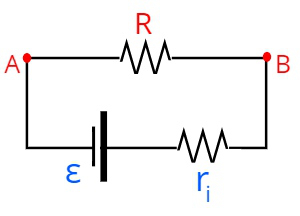ε – electromotive force

According to the characteristic equation of generators , the useful electrical potential applied to resistor R is equal to the difference between the electromotive force and the electrical voltage dissipated by the operation of the generator itself, due to the joule effect . This dissipated voltage, in turn, is equal to the product of internal resistance and electric current , as stated in Ohm’s first law .

Once the useful voltage is applied to resistor R, we say that this voltage is equivalent to that formed between the resistor terminals, check: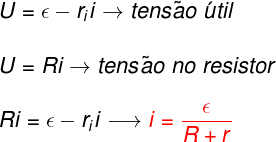When we isolate the electric current variable, i, we find the expression highlighted in red, which is a simplified form of Pouillet’s law, for a circuit that contains only generators and resistors.

The following figure shows a more elaborate electrical circuit that contains a generator , a receiver , and a resistor.

What defines, in this case, what is a generator and what is a receiver is the direction of the electric current : when the electric current crosses from the largest terminal (larger vertical bar) to the smallest terminal (smaller vertical bar), it is a receiver, otherwise the element is a generator.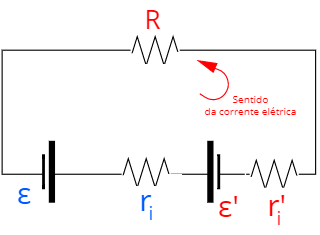If there are receivers , in addition to generators and resistors, in the circuit, Pouillet’s law must take into account the counter- electromotive force consumed by the receivers, note:ε’ – counter electromotive force

i – internal resistance of the generator

i – internal resistance of the receiver

R – circuit resistance

The most general representation of Pouillet’s law is written in the form of summations , considering that, in a mesh, it is possible that there are series associations of generators and resistors.

To calculate electric current using Pouillet’s law, we add all the electromotive forces from the generators, then subtract from the sum of all the counter electromotive forces. The result obtained must then be divided by the sum of all electrical resistances in the loop. Check out in this figure the general formula of Pouillet’s law: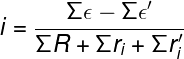## Exercises on Pouillet’s Law

Question 1) An electric motor with an electromotive force of 7.0 V and an internal resistance of 1.0 Ω is connected to an electric generator of 12.0 V and an internal resistance of 1.0 Ω. Determine the magnitude of the electric current formed in this motor.

a) 1.5 A

b) 2.5 A

c) 0.5 A

d) 1.9 A

e) 0.7 A

Template: letter b

Resolution:

The statement speaks of an electric motor, which is a receiver, connected directly to a real generator. In this way, we will use Pouillet’s law, assuming that there is no additional external resistance (R) in the circuit, in addition to the internal resistances of the generator and receiver: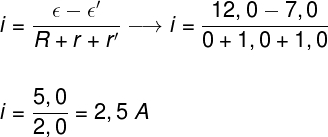Question 2) Four batteries of 1.5 V and internal resistance of 0.25 Ω, are connected in series and then connected to a 4.0 V electric motor with back electromotive force. Knowing that the electric current formed in the motor is equal to 0.5 A, determine the internal resistance of this motor.

a) 3 Ω

b) 5 Ω

c) 2 Ω

d) 13 Ω

e) 15 Ω

Template : letter a

Resolution:

We are going to use Pouillet’s law to solve this exercise, for that, we should note that, when connected in series, the four batteries will produce an electromotive force of 6.0 V, and the equivalent resistance of this association will be equal to 1.0 Ω, note the calculations:Question 3) Two ideal generators of 8.0 V, of electromotive force, are associated in series, being then connected to an electric motor, of back electromotive force equal to 8.0 V and internal resistance of 2.5 Ω. Determine the magnitude of the electric current flowing through the electric motor.

a) 2.8 A

b) 3.2 A

c) 3.5 A

d) 2.4 A

e) 1.6 A

Template: letter b

Resolution :

To solve the exercise, we will use Pouillet’s law, for that, we must note that the total electromotive force is 16.0 V, while the counter electromotive force is 8.0 V. In this way, we just have to solve the calculation shown below :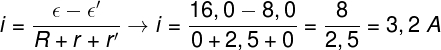Check Also
Close